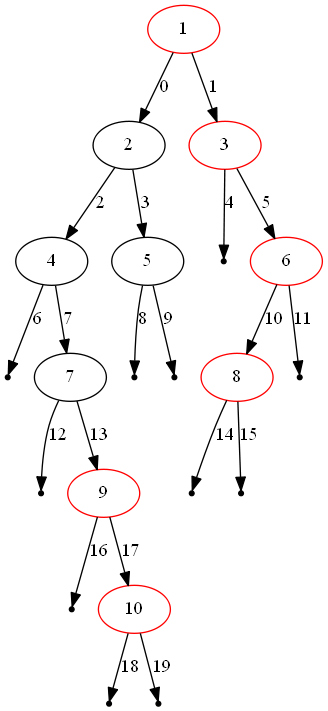## 199. Binary Tree Right Side View

Given a binary tree, imagine yourself standing on the right side of it, return the values of the nodes you can see ordered from top to bottom.

For example:
Given the following binary tree,

```   1            <---
/   \
2     3         <---
\     \
5     4       <---
```

You should return `[1, 3, 4]`.

Credits:
Special thanks to @amrsaqr for adding this problem and creating all test cases.

b'
\n\n

#### Initial Thoughts

\n

Because the tree topography is unknown ahead of time, it is not possible to\ndesign an algorithm that visits asymptotically fewer than nodes.\nTherefore, we should try to aim for a linear time solution. With that in\nmind, let\'s consider a few equally-efficient solutions.

\n

#### Approach #1 Depth-First Search [Accepted]

\n

Intuition

\n

We can efficiently obtain the right-hand view of the binary tree if we visit\neach node in the proper order.

\n

Algorithm

\n

One of the aforementioned orderings is defined by a depth-first search in\nwhich we always visit the right subtree first. This guarantees that the first\ntime we visit a particular depth of the tree, the node that we are visiting\nis the rightmost node at that depth. Therefore, we can store the value of the\nfirst node that we visit at each depth, ultimately generating a final array\nof values once we know exactly how many layers are in the tree.

\n\n

The figure above illustrates one instance of the problem. The red nodes\ncompose the solution from top to bottom, and the edges are labelled in order\nof visitation.

\n\n

Complexity Analysis

\n
\n
• \n

Time complexity : .

\n

Because a binary tree with only child pointers is directed acyclic graph\nwith only one source node, a traversal of the tree from the root will visit\neach node exactly once (plus a sublinear amount of leaves, represented as\n`None`). Each visitation requires only work, so the while loop\nruns in linear time. Finally, building the array of rightmost values is\nheight of the tree because it is not possible for a\nright-hand view of the tree to contain more nodes than the tree itself.

\n
• \n
• \n

Space complexity : .

\n

At worst, our stack will contain a number of nodes close to the height of\nthe tree. Because we are exploring the tree in a depth-first order, there\nare never two nodes from different subtrees of the same parent node on the\nstack at once. Said another way, the entire right subtree of a node will be\nvisited before any nodes of the left subtree are pushed onto the stack. If\nthis logic is applied recursively down the tree, it follows that the stack\nwill be largest when we have reached the end of the tree\'s longest path\n(the height of the tree). However, because we know nothing about the tree\'s\ntopography, the height of the tree may be equivalent to , causing the\nspace complexity to degrade to .

\n
• \n
\n
\n

#### Approach #2 Breadth-First Search [Accepted]

\n

Intuition

\n

Much like depth-first search can guarantee that we visit a depth\'s rightmost\nnode first, breadth-first search can guarantee that we visit it last.

\n

Algorithm

\n

By performing a breadth-first search that enqueues the left child before the\nright child, we visit each node in each layer from left to right. Therefore,\nby retaining only the most recently visited node per depth, we will have\nthe rightmost node for each depth once we finish the tree traversal. The\nalgorithm is unchanged, other than swapping out the stack for a\n`deque`1 and removing the containment check before assigning into\n`rightmost_value_at_depth`.

\n\n

The figure above illustrates the same instance as before, but solved via\nbreadth-first search. The red nodes compose the solution from top to bottom,\nand the edges are labelled in order of visitation.

\n\n

Complexity Analysis

\n
\n
• \n

Time complexity : .

\n

The differences itemized in the Algorithm section do not admit\ndifferences in the time complexity analysis between the bread-first and\ndepth-first search approaches.

\n
• \n
• \n

Space complexity : .

\n

Because breadth-first search visits the tree layer-by-layer, the queue\nwill be at its largest immediately before visiting the largest layer. The\nsize of this layer is in the worst case (a complete binary\ntree).

\n
• \n
\n
\n

Footnotes

\n
\n

Analysis written by: @emptyset

\n
\n
\n
\n
1. \n

The\n`deque`\ndatatype from the\n`collections` module\nsupports constant time append/pop from both the head and the tail. If we were\nto use a Python `list`, it would cost us time to remove its head via\n`list.pop(0)`.\xc2\xa0\xe2\x86\xa9

\n
2. \n
\n
\n
'